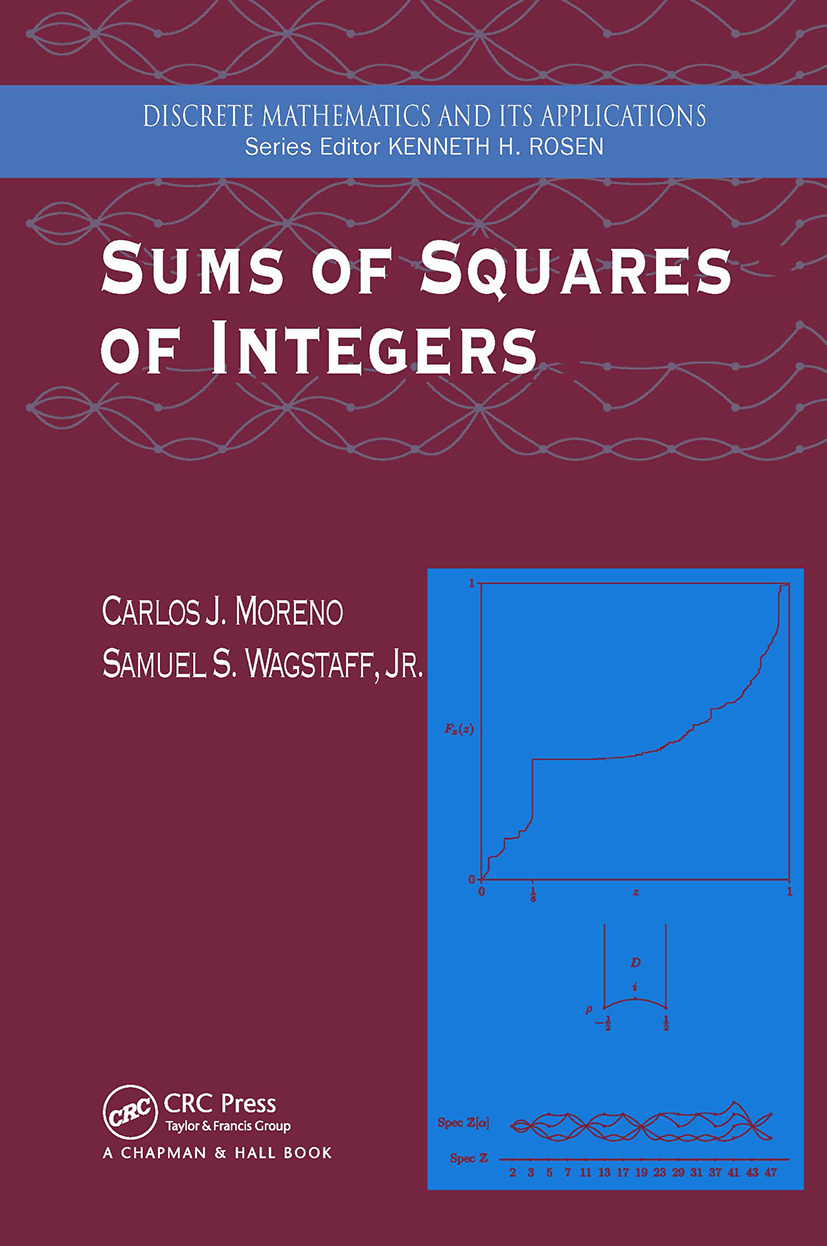Sums of Squares of Integers

1st Edition

Chapman and Hall/CRC

368 pages

Purchasing Options:\$ = USD
Paperback: 9780367391614
pub: 2019-09-05
SAVE ~\$14.99
\$74.95
\$59.96
x
Hardback: 9781584884569
pub: 2005-12-09
SAVE ~\$29.00
\$145.00
\$116.00
x
eBook (VitalSource) : 9780429124419
pub: 2005-12-09
from \$37.48

FREE Standard Shipping!

Description

Sums of Squares of Integers covers topics in combinatorial number theory as they relate to counting representations of integers as sums of a certain number of squares. The book introduces a stimulating area of number theory where research continues to proliferate. It is a book of "firsts" - namely it is the first book to combine Liouville's elementary methods with the analytic methods of modular functions to study the representation of integers as sums of squares. It is the first book to tell how to compute the number of representations of an integer n as the sum of s squares of integers for any s and n. It is also the first book to give a proof of Szemeredi's theorem, and is the first number theory book to discuss how the modern theory of modular forms complements and clarifies the classical fundamental results about sums of squares.

The book presents several existing, yet still interesting and instructive, examples of modular forms. Two chapters develop useful properties of the Bernoulli numbers and illustrate arithmetic progressions, proving the theorems of van der Waerden, Roth, and Szemeredi. The book also explains applications of the theory to three problems that lie outside of number theory in the areas of cryptanalysis, microwave radiation, and diamond cutting. The text is complemented by the inclusion of over one hundred exercises to test the reader's understanding.

Introduction. Elementary Methods. Bernoulli Numbers. Examples of Modular Forms. Hecke's Theory of Modular Forms. Representation of Numbers as Sums of Squares. Arithmetic Progressions. Applications. References. Index.

Moreno, Carlos J.; Wagstaff, Jr.